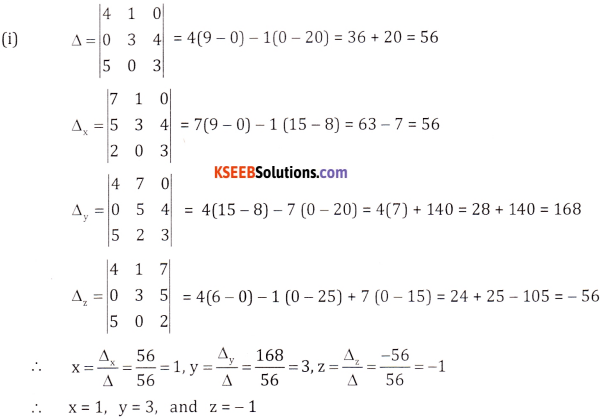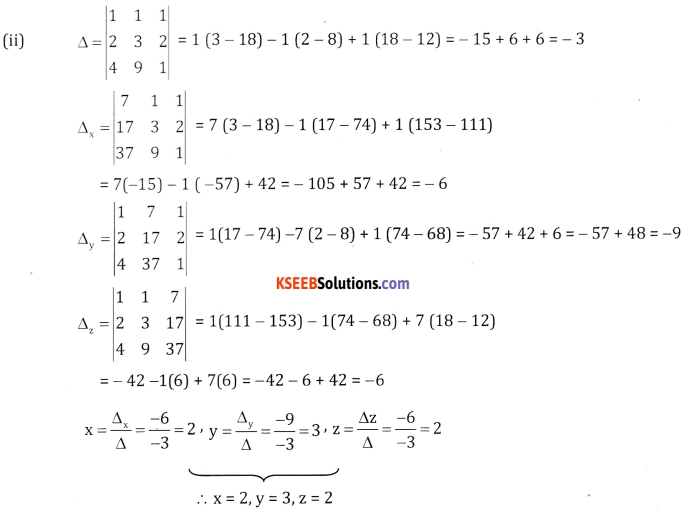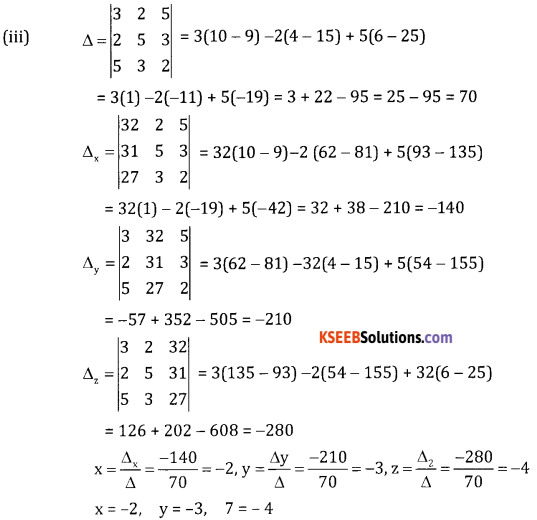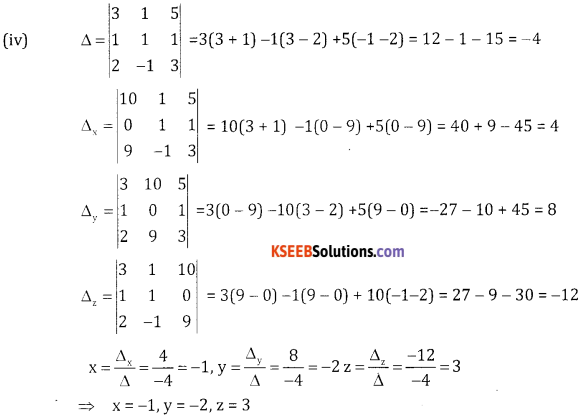# 2nd PUC Basic Maths Question Bank Chapter 1 Matrices and Determinants Ex 1.5

Students can Download Basic Maths Exercise 1.5 Questions and Answers, Notes Pdf, 2nd PUC Basic Maths Question Bank with Answers helps you to revise the complete Karnataka State Board Syllabus and score more marks in your examinations.

## Karnataka 2nd PUC Basic Maths Question Bank Chapter 1 Matrices and Determinants Ex 1.5

Part – A

2nd PUC Basic Maths Matrices and Determinants Ex 1.5 One Mark Questions and Answers

Question 1.
Solve the following by cramer’s rule
(I) 2x + 3y = 11 ; x – y – 3 = 0
(ii) 3x + 4y = 7 ; 7x = y + 6
(iii) 3x + 2y = 8  ;4x – 3y = 5
(iv) 2x + y = 1 ; x – 3y = 4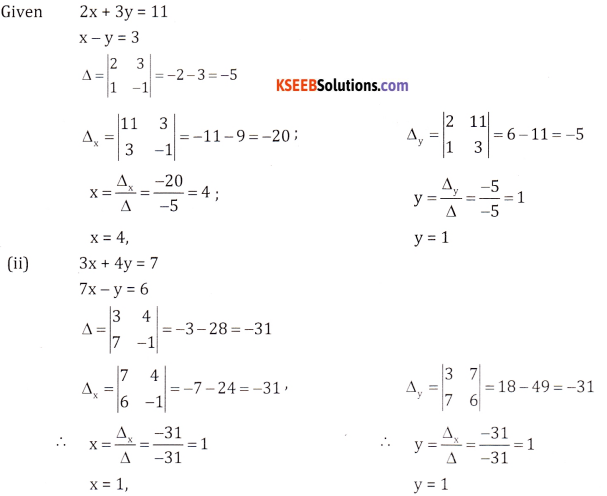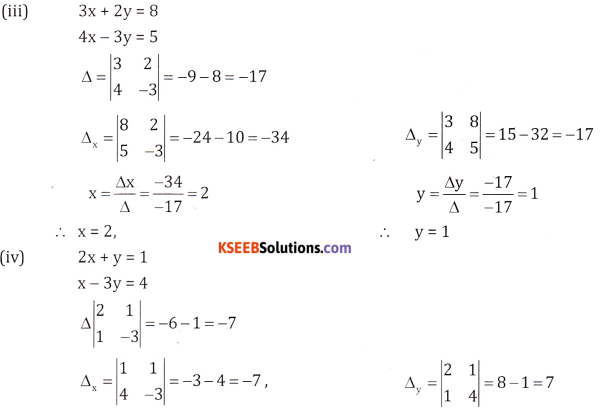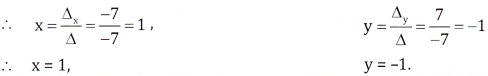Question – 2.
Sove the following by cramer’s rule
(i) 4x + y = 7
3y + 4z = 5
3z + 5x = 2

(ii) x + y + z = 7
2x + 3y + 2z = 17
4x + 9y + z = 37

(iii) 3x + 2y + 5z = 32
2x + 5y + 3z = 31
5x + 3y + 2z = 27

(iv) 3x + y + 5z =10
x + y + z = 0
2x – y + 3z = 9